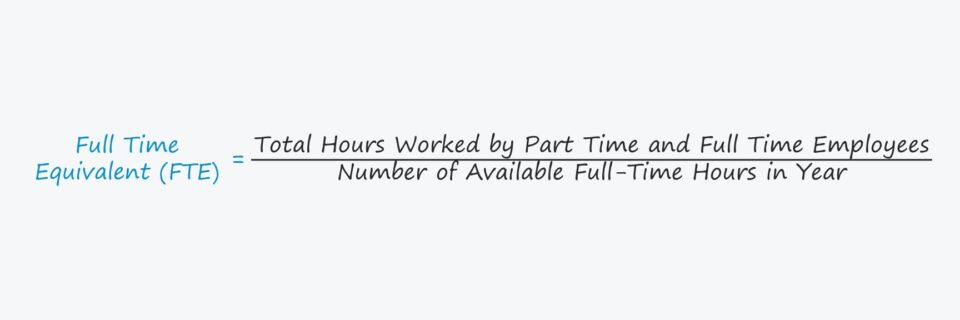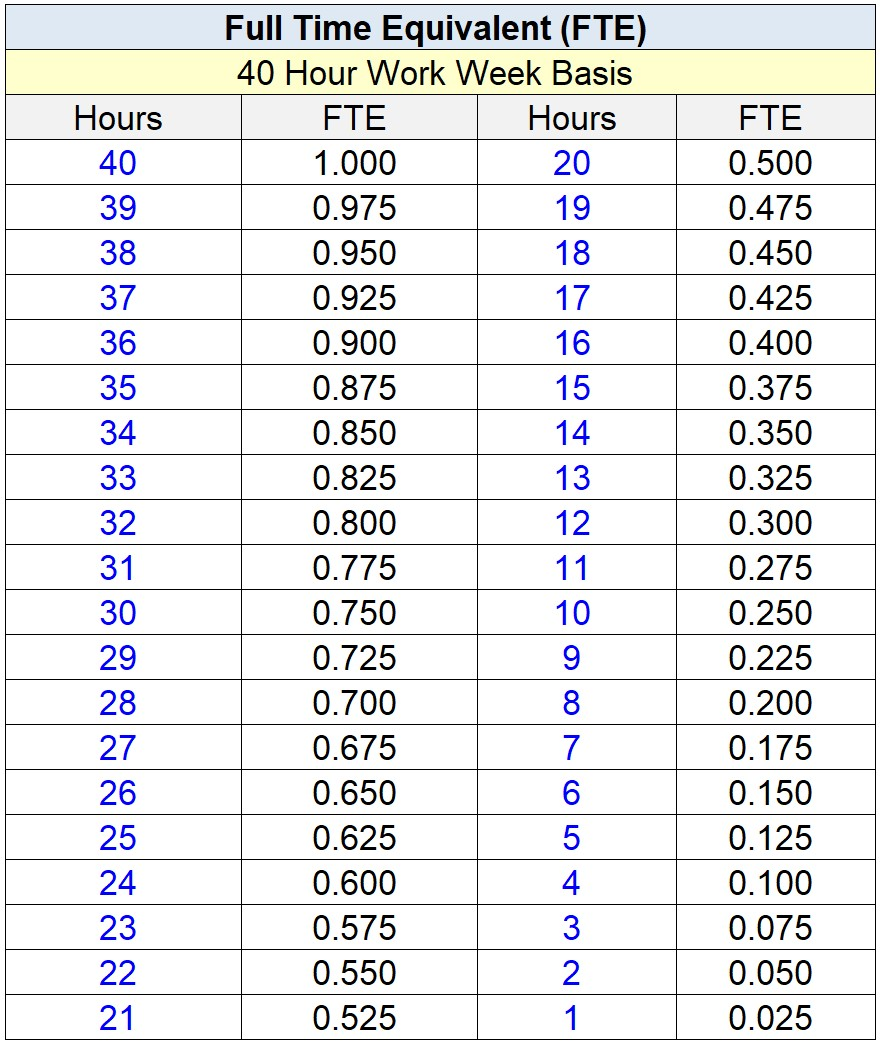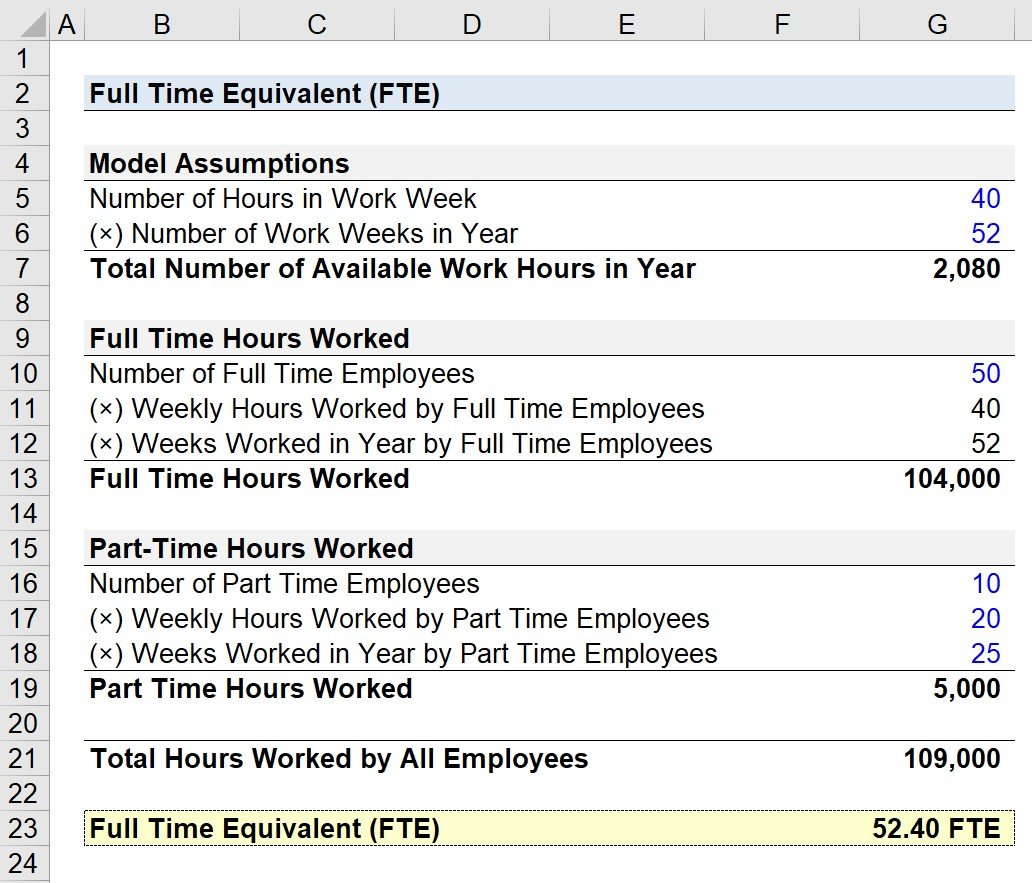Welcome to Wall Street Prep! Use code at checkout for 15% off.# Full Time Equivalent (FTE)

Guide to Understanding Full Time Equivalent (FTE)## How to Calculate Full Time Equivalent (FTE)?

FTE, an abbreviation for “full time equivalent”, counts the approximate number of full-time employees employed by a specific company, but with the inclusion of part-time employees.

Employers can use FTE to evaluate their hiring capacity and current payroll, as well as for reporting purposes related to labor laws and employee benefit programs.

The FTE calculation counts part-time employees as a fraction of a full-time employee, most often on the basis of the number of hours worked.

The baseline in order to qualify as 1.0 unit of a full-time employee is set at 40 hours, so a part-time employee that worked 20 hours would be marked as a 0.5 FTE.

• 40-Hour Work Week → 1.0 FTE
• 20-Hour Work Week → 0.5 FTE

Calculating the full time equivalent (FTE) is a four-step process:

1. Count the Number of Hours Worked by Part-Time Employees
2. Count the Number of Hours Worked by Full-Time Employees
3. Add the Number of Hours Worked by Both Part-Time and Full-Time Employees
4. Divide the Total Hours Worked by All Employees by the Number of Available Full-Time Hours in a Year (2,080 Hours)

## Full Time Equivalent Formula (FTE)

The formula for calculating the full time equivalent (FTE) on an annual basis is as follows.

Full-Time Equivalent (FTE) = Total Hours Worked by Part-Time and Full-Time Employees ÷ Number of Available Full-Time Hours in Year

The total hours worked is the sum of the number of hours worked by part-time and full-time employees, whereas the number of available full-time hours in a year is most frequently 2,080.

## Full Time Equivalent Chart: 40-Hour Work Week

The chart below displays the number of weekly hours worked with the corresponding FTE under the standard 40 work-week.## FTE Calculator

We’ll now move to a modeling exercise, which you can access by filling out the form below.Submitting...

## 1. Employee Assumptions

Suppose a company currently has 50 full-time employees on its payroll, along with 10 part-time employees.

The full-time employees work 40 hours per week and 52 weeks out of the year, meaning that the number of full-time hours worked on an annualized basis is 104,000 hours.

• Full Time Hours Worked = 50 × 40 × 52 = 104,000

The part-time employees, on the other hand, work 20 hours per week and 25 weeks out of the year.

Using the same formula, the number of part-time hours worked amounts to 5,000 hours per year.

• Part Time Hours Worked = 10 × 20 × 25 = 5,000

By adding the full-time and part-time hours worked, we can calculate the total number of hours worked by all employees for the year as 109,000.

• Total Hours Worked by All Employees = 104,000 + 5,000 = 109,000

The 109,000 total hours worked must be divided by the total number of available work hours in a year, or 2,080 hours.

• Total Number of Available Work Hours in Year = 40 × 52 = 2,080

## 2. FTE Calculation Example

With the required inputs in place, we can arrive at approximately 52.40 FTEs by dividing the total hours worked by all employees by the total number of available work hours in a year.

• Full Time Equivalent (FTE) = 109,000 ÷ 2,080 = 52.40 FTEs

In our hypothetical scenario, our company has the equivalent of 52.40 full-time employees.Step-by-Step Online Course

### Everything You Need To Master Financial Modeling

Enroll in The Premium Package: Learn Financial Statement Modeling, DCF, M&A, LBO and Comps. The same training program used at top investment banks.